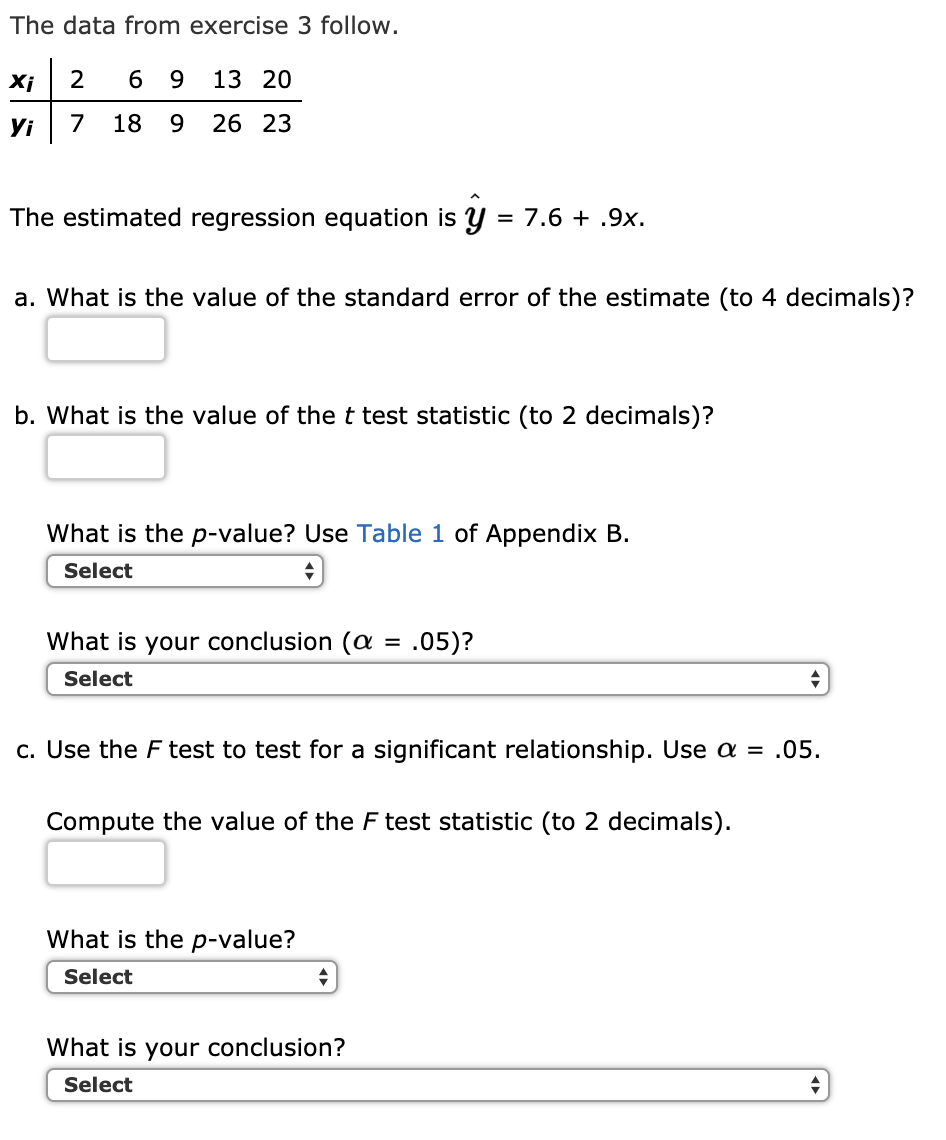# The data from exercise 3 followxi2 6 9 13 20Vi7 18 9 26 23The estimated regression equation is y- 7.6 + .9x.a. What is the value of the standard error of the estimate (to 4 decimals)?b. What is the value of the t test statistic (to 2 decimals)?What is the p-value? Use Table 1 of Appendix B.SelectWhat is your conclusion (a.05)?SelectC. Use the F test to test for a significant relationship. Use α-.05.Compute the value of the F test statistic (to 2 decimals)What is the p-value?SelectWhat is your conclusion?Select

Question

The data from exercise 3 follow.

 xi 2 6 9 13 20 yi 7 18 9 26 23

The estimated regression equation is  = 7.6 + .9x.

1. What is the value of the standard error of the estimate (to 4 decimals)?

2. What is the value of the t test statistic (to 2 decimals)?

What is the p-value? Use Table 1 of Appendix B.
Selectless than .01between .01 and .02between .02 and .05between .05 and .10between .10 and .20between .20 and .40greater than .40Item 3

What is your conclusion ( = .05)?
SelectConclude a significant relationship exists between x and yCannot conclude a significant relationship exists between x and yItem 4

3. Use the F test to test for a significant relationship. Use  = .05.

Compute the value of the F test statistic (to 2 decimals).

What is the p-value?
Selectless than .01between .01 and .025between .025 and .05between .05 and .10greater than .10Item 6

SelectConclude a significant relationship exists between x and yCannot conclude a significant relationship exists between x and yhelp_outlineImage TranscriptioncloseThe data from exercise 3 follow xi2 6 9 13 20 Vi7 18 9 26 23 The estimated regression equation is y- 7.6 + .9x. a. What is the value of the standard error of the estimate (to 4 decimals)? b. What is the value of the t test statistic (to 2 decimals)? What is the p-value? Use Table 1 of Appendix B. Select What is your conclusion (a.05)? Select C. Use the F test to test for a significant relationship. Use α-.05. Compute the value of the F test statistic (to 2 decimals) What is the p-value? Select What is your conclusion? Select fullscreen
check_circle

Step 1

1.

The given estimated regression equation is y-hat = 7.6 + 0.9 x and the value of n is 5.

Required calculations for finding standard error are as follows:

Step 2

The value of standard error is as follows:

Step 3

2.

Hypotheses:

Null hypothesis: There no significant relationship exists between x and y.

Alternative hypothesis: There is a significant relations...

### Want to see the full answer?

See Solution

#### Want to see this answer and more?

Solutions are written by subject experts who are available 24/7. Questions are typically answered within 1 hour.*

See Solution
*Response times may vary by subject and question.
Tagged in

### Statistics